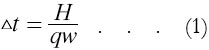# Temperature Rise in Utah Projectile

(Reproduced from "Zero to Eighty" pp.326 by EF Northrup)

It is estimated that the heat produced in the projectile by the high-frequency currents will be about twenty-five per cent greater than the equivalent kinetic energy of motion imparted by the thrust. The kinetic energy has been given as 41 x 105 kilogram meters1 which is equivalent to approximately 96 x 105 small calories. Adding twenty-five per cent brings the total heat energy to 12 x 100 calories.

Assuming that seventy-five kilograms of the projectile are available for absorbing heat, and that the average specific heat is about 0.25 calories per gram per degree Centigrade, we find the temperature rise from the following formula:where dt is the temtemperature rise in degrees Centigrade, H the heat absorbed in calories, q the specific heat of the absorbing material, and w the weight of the material in grams.

By substitution in Equation 1, the temperature rise is found to be 640° C.

Actually, however, the interior of the projectile will be raised in temperature very little, because the stored-up heat will be carried away by the sodium-potassium alloy2 and by convection and radiation.

1 See pp. 147-148 of the narrative.
2 See pp. 138-140 of the narrative.

 < Previous Page 13 of 17 Next >